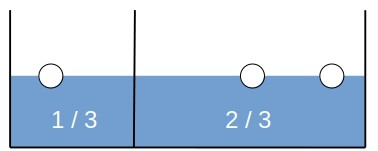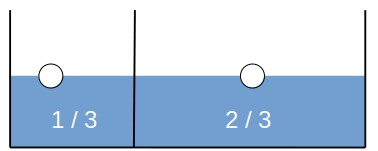## 1. Introduction

In this tutorial, we’ll talk about the Monty Hall Problem. We’ll explain its solution and talk about why people usually fail to solve the problem correctly.

## 2. The Monty Hall Problem

The Monty Hall Problem (or the Monty Hall Dilemma) is a math puzzle notorious for its counter-intuitive solution. It’s adapted from the TV show “Let’s Make a Deal” and is usually stated like this:

A guest on a TV show chooses between three doors. Randomly placed behind one of them, there’s a prize. The other two hide a goat. After the guest selects a door, the host, Monty Hall, opens one of the two unchosen ones. Then, he offers the guest a chance to change his choice. Should the contestant stick to his initial choice or switch to the other door?

In other words, which action has a greater probability of winning the prize?

## 3. The Wrong Solution

Most people answer that they would stick to their initial choice.

The reasoning is usually that all three doors are equally likely to hide the prize. After the host opens one of the two unchosen doors (and Monty Hall will always open a door with a goat behind it), we’re left with two options that initially had the same probability of success. The ratio of their probabilities doesn’t change because the door the host opens tells us nothing about which of the other two doors hides the prize and doesn’t change where the prize is. So, the probability of each is. Since it doesn’t matter which door we choose at this stage, we may as well stay with our initial choice.

However, the reasoning is flawed. Not just that the probabilities aren’t equal, but switching to the other door has a chance ofto win the prize!

## 4. Intuitive Explanation

Let’s try and explain the solution in lay terms before deriving it formally using the probability theory.

Since the prize is placed randomly behind one of them, when we make our choice, we assignof our total probability to that door. Likewise, the chance that the prize isn’t behind the chosen door, i.e., that it’s behind one of the other two doors, must be.

Now, let’s imagine we have 1 liter of water representing the total probability we can assign to the doors. In addition, we have a rectangular container with three balls denoting the three doors. Choosing a door at the start is like dividing the container with a solid obstacle so that the ball representing our door is in the smaller part, and pouringandof water into the smaller and bigger parts, respectively:### 4.1. What Happens After Monty Hall Opens a Door?

When Monty Hall opens one of the other two doors and shows there’s a goat behind it, he takes that door’s ball out of the part containing 2/3 of the total volume. So, now we have our initial door’s ball  floating in 1/3, and the remaining unselected door in 2/3 of the water:Since the water represents the probability, the chance that the other door hides the prize is.

### 4.2. Why Don’t We Redistribute Probability Between the Remaining Two Doors?

We may ask why Monty couldn’t, while taking out the ball, pour some of the water into our door’s part so that the two contain the same quantity of water.

The water in the larger part is the probability that we were initially wrong. The water in the smaller part represents the probability that the chosen door hides the prize. Were we to mix waters from the two parts after the choice, we’d try to combine probabilities of different meanings (that we chose the right door and that we didn’t) but represent only one (that we were right) with the mixture as a whole.

Since Monty’s choice doesn’t change the correctness of our initial decision, we still have the chance ofto win the prize if we stick to it. But, the probability associated with the remaining unchosen door changes because once Monty opens a door, the probability ofconcentrates on the one left: we don’t allocate any probability that the prize is behind the opened door since we see a goat was behind it.

## 5. The Classical Approach

We can formally solve the problem using the probability theory. The classical approach constructs the event space and determines the success probabilities of two strategies by adding their constituent elementary events’ probabilities.

### 5.1. Before Monty Hall Opens a Door

There are three possible arrangements at the start:where,, andare the doors,denotes the prize, andrepresents a goat.

Since we have three doors to choose from, there are nine possible “worlds” depending on our initial choice (). All of them are equally probable because all the decisions up to this point were random. Let’s denote the probability of a row withand see what the space of possible worlds is like:### 5.2. Monty Hall Opens a Door

Monty Hall doesn’t open the door with the prize. So, if the prize isn’t behind the door we chose, Monty Hall’s choice isn’t random: he opens the door hiding the goat with the probability of. In contrast, his choice is random if our initial guess was correct. He’ll open any of the two remaining doors with the probability of. Let’s denote his choice withand see how it affects the space of possible events and their probabilities:### 5.3. To Switch or Not to Switch?

That’s our event space. It containselementary events, not all equally likely. To find the probability of the event“The prize is behind the initially chosen door“, we sum the probabilities of the rows in which the prize is behind the door denoted by. Those are:So, the probability ofis:Therefore, if we stick to the initial choice, we have the probability ofto win the prize. However, if we switch, the probability of winning rises to.

The key observation to arrive at the correct conclusion is that Monty Hall’s choice isn’t random if we don’t select the door with the prize. Without that observation, we’d treat all the rows as equally likely and compute, erroneously concluding that the two strategies are the same.

## 6. The Bayesian Approach

The classical approach with the event space gives us the correct solution. However, the proof is rather long. We can write a shorter one if we use Bayes’ Theorem:

(1)whereis the hypothesis andis the evidence supporting (or refuting it).is known as the prior probability of, andis its posterior probability. The application of rule (\eqref{eq:bayes}) is called the Bayesian update because it changes our prior beliefs (based on the evidence gathered).

### 6.1. Notation

We” define three hypotheses:We’ll denote our initial choice asand define the eventas “Monty Hall opens door“, whereand. Of interest is the posterior, that is, the probability that the prize is behind the door we choose at the start of the game:

(2)Let’s determine each component in Equation (2).

### 6.2. The Priors

Since the doors are equally likely to conceal the prize, our priors will be uniform:### 6.3. The Conditional Probability

The conditional probabilityisbecause Monty Hall opens one of the other two doors at random if our initial guess is correct.

### 6.4. The Probability of Monty Hall’s ChoiceTo find the posterior probability, we also need. We compute it using the law of total probability:Since, wheredenotes the door we didn’t choose and Monty Hall didn’t open (), we have:So:### 6.5. The Posterior

Finally, we calculate the posterior probabilityusing Equation (2):So, the probability that the prize is behind the door we selected at the start is. The probability that the prize is behind the other door isbecause only two hypotheses remain after Monty Hall opens door:and, and their probabilities, therefore, sum to.

## 7. Why People Get the Monty Hall Problem Wrong

Most people find the solution and proofs counter-intuitive. Even though the problem was first presented (and solved) in 1975 by a Berkeley statistician Steve Selvin, the problem attracted mass attention in 1990 when Marilyn vos Savant explained in her column in the Parade magazine why the correct answer was to switch the door. She received thousands of letters from readers claiming she was wrong, many of whom even had PhDs and worked in mathematics departments at US universities and research facilities.

That’s when psychologists got interested in the problem. They found that most people would stick to their initial choice and not understand why it was wrong. Even proficiency in math isn’t a guarantee that a person will solve the problem correctly.

A possible explanation is that people experience regret more intensely if they lose the prize by switching from the door hiding it than if they stick to the one with a goat. Even when they understand the solution, the anticipated regret they associate with the loss through their actions is stronger than the mathematical proof that switching is a rational choice. There are other theories why humans fail to identify the right choice, and it seems that our common sense and intuition make us susceptible to fallacies of statistical reasoning.

## 8. Conclusion

In this article, we presented the Monty Hall Problem. We offered an intuitive explanation of why switching is a better strategy after the host opens a door. Additionally, we gave two proofs: one by constructing and enumerating the event space and the other using Bayes’ Theorem.

Even though the solution isn’t controversial mathematically, the Monty Hall Problem still attracts attention. It’s interesting to study from the psychological perspective as it sheds light on human statistical intuition and biases.